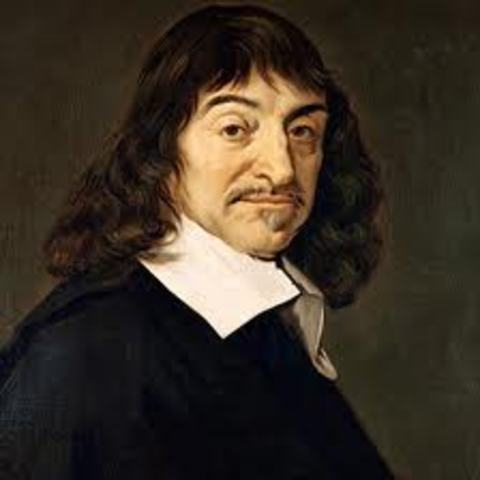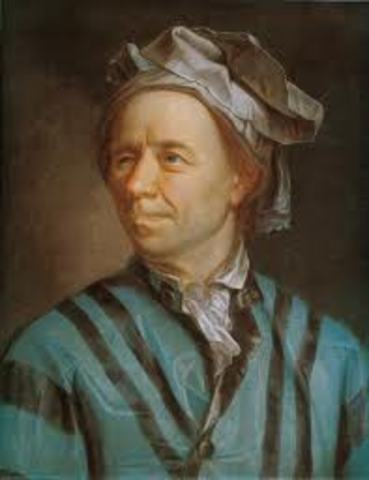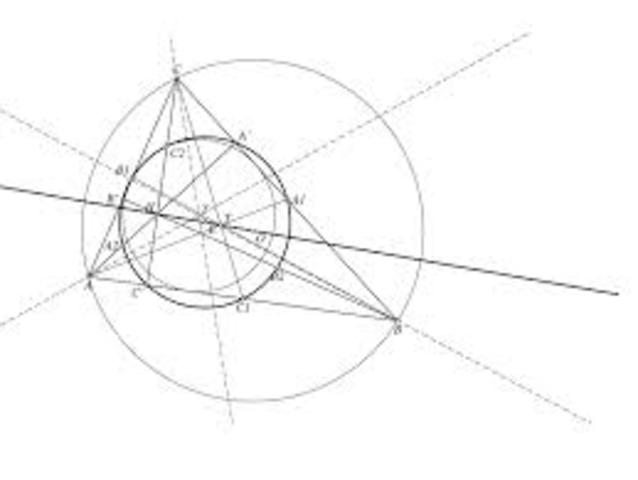By GHHSWH1
• René DescartesRené Descartes proposed the idea of "La Géométrie". He stated that each point in two dimensions can be described by two numbers on a plane, one giving the point’s horizontal location and the other the vertical location. These have come to be known as Cartesian coordinates. He used perpendicular lines (or axes), crossing at a point called the origin, to measure the horizontal (x) and vertical (y) locations, both positive and negative, and dividing the plane into four separate quadrants.
• Bonaventura CavalieriBonaventura Cavalieri’s method of indivisibles rests on the assumption that a line is composed of an infinite number of successive points, a plane figure of an infinite number of parallel line segments, and a solid body of an infinite number of parallel plane areas. Cavalieri used Descartes’ idea of a coordinate plane and began finding points and segments on the plane.
• Leonhard EulerLeonhard Euler brought together everything that was then known of calculus and geometry. These works divorced the subject from its geometrical origins and shaped its direction for the next 50 years. He also popularized the use of many mathematical symbols. He did work with equations and formulas on coordinate planes that both Descartes and Cavalieri did work with. He began using coordinate planes with shapes, which is what we do a lot of in geometry today.
• Geometry TodayToday we use coordinate planes in geometry class. We use planes to find area, distance, midpoint, and perimeter of shapes. They can be used for plotting points, architectural layouts, and finding places on maps. Architects use coordinate planes on a daily basis by drawing their layouts on the grid for a model of their designs.